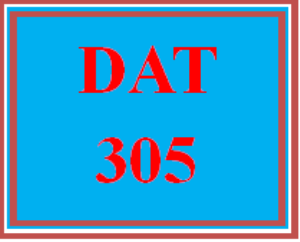# DAT 305 Week 5 Apply - Wk 5 Quiz

PLDZ-12298 In Stock
\$ 8.00 USD
Description

***************************************************************

https://uopcourse.com/category/dat-305/

~~~~~~~~~~~~~~~~~~~~~~~~~~~~~~~~~~~~~~~~~~~~~~~~~

https://uopcourse.com/

***************************************************************

DAT 305 Week 5 Apply – Wk 5 Quiz

Given the previous Java implementation of an adjacency list representation of a directed graph, what is the runtime complexity of computing the out-degree of every vertex?

a. O(V2)

b. O(V*E)

c. O(V + E)

d. O(V)

Given the previous Java implementation of an adjacency list representation of a directed graph, what is the runtime complexity of computing the in-degree of every vertex?

a. O(V2)

b. O(V*E)

c. O(V + E)

d. O(V)

Given the previous Java implementation of an adjacency matrix representation of a directed graph, what is the runtime complexity of counting the number of edges of the graph?

a. O(V + E)

b. O(V2)

c. O(V)

d. O(V*E)

A vertex u of a directed graph can end up in a depth-first tree containing only u, even though u has both outward and inward edges.

a. True

b. False

If vertices u and v are in different trees of the depth-first forest, then u and v must not be connected.

a. True

b. False

A depth-first tree is always different from a breadth-first tree rooted at the same vertex for the same graph G.

a. True

b. False

Using which one of the following data structures can we implement Dijkstra’s shortest path algorithm on unweighted graphs so that it runs in linear time?

a. Binary Tree

b. Queue

c. Heap

d. Stack

Given a directed graph with negative edge weights, Dijkstra’s algorithm is capable of finding the shortest path between two nodes.

a. True

b. False

Which algorithm design paradigm does the Floyd-Warshall algorithm follow?

a. Divide and conquer

b. Greedy

c. Prune and search

d. Dynamic programming

Recent Reviews Write a Review
0 0 0 0 reviews